# Fraction calculator

The calculator performs basic and advanced operations with fractions, expressions with fractions combined with integers, decimals, and mixed numbers. It also shows detailed step-by-step information about the fraction calculation procedure. Solve problems with two, three, or more fractions and numbers in one expression.

## Result:

### 63/5 - 43/4 = 37/20 = 1 17/20 = 1.85

Spelled result in words is thirty-seven twentieths (or one and seventeen twentieths).

### How do you solve fractions step by step?

1. Conversion a mixed number 6 3/5 to a improper fraction: 6 3/5 = 6 3/5 = 6 · 5 + 3/5 = 30 + 3/5 = 33/5

To find new numerator:
a) Multiply the whole number 6 by the denominator 5. Whole number 6 equally 6 * 5/5 = 30/5
b) Add the answer from previous step 30 to the numerator 3. New numerator is 30 + 3 = 33
c) Write a previous answer (new numerator 33) over the denominator 5.

Six and three fifths is thirty-three fifths
2. Conversion a mixed number 4 3/4 to a improper fraction: 4 3/4 = 4 3/4 = 4 · 4 + 3/4 = 16 + 3/4 = 19/4

To find new numerator:
a) Multiply the whole number 4 by the denominator 4. Whole number 4 equally 4 * 4/4 = 16/4
b) Add the answer from previous step 16 to the numerator 3. New numerator is 16 + 3 = 19
c) Write a previous answer (new numerator 19) over the denominator 4.

Four and three quarters is nineteen quarters
3. Subtract: 33/5 - 19/4 = 33 · 4/5 · 4 - 19 · 5/4 · 5 = 132/20 - 95/20 = 132 - 95/20 = 37/20
For adding, subtracting, and comparing fractions, it is suitable to adjust both fractions to a common (equal, identical) denominator. The common denominator you can calculate as the least common multiple of both denominators - LCM(5, 4) = 20. In practice, it is enough to find the common denominator (not necessarily the lowest) by multiplying the denominators: 5 × 4 = 20. In the next intermediate step, the fraction result cannot be further simplified by canceling.
In words - thirty-three fifths minus nineteen quarters = thirty-seven twentieths.

#### Rules for expressions with fractions:

Fractions - use the slash “/” between the numerator and denominator, i.e., for five-hundredths, enter 5/100. If you are using mixed numbers, be sure to leave a single space between the whole and fraction part.
The slash separates the numerator (number above a fraction line) and denominator (number below).

Mixed numerals (mixed fractions or mixed numbers) write as non-zero integer separated by one space and fraction i.e., 1 2/3 (having the same sign). An example of a negative mixed fraction: -5 1/2.
Because slash is both signs for fraction line and division, we recommended use colon (:) as the operator of division fractions i.e., 1/2 : 3.

Decimals (decimal numbers) enter with a decimal point . and they are automatically converted to fractions - i.e. 1.45.

The colon : and slash / is the symbol of division. Can be used to divide mixed numbers 1 2/3 : 4 3/8 or can be used for write complex fractions i.e. 1/2 : 1/3.
An asterisk * or × is the symbol for multiplication.
Plus + is addition, minus sign - is subtraction and ()[] is mathematical parentheses.
The exponentiation/power symbol is ^ - for example: (7/8-4/5)^2 = (7/8-4/5)2

#### Examples:

subtracting fractions: 2/3 - 1/2
multiplying fractions: 7/8 * 3/9
dividing Fractions: 1/2 : 3/4
exponentiation of fraction: 3/5^3
fractional exponents: 16 ^ 1/2
adding fractions and mixed numbers: 8/5 + 6 2/7
dividing integer and fraction: 5 ÷ 1/2
complex fractions: 5/8 : 2 2/3
decimal to fraction: 0.625
Fraction to Decimal: 1/4
Fraction to Percent: 1/8 %
comparing fractions: 1/4 2/3
multiplying a fraction by a whole number: 6 * 3/4
square root of a fraction: sqrt(1/16)
reducing or simplifying the fraction (simplification) - dividing the numerator and denominator of a fraction by the same non-zero number - equivalent fraction: 4/22
expression with brackets: 1/3 * (1/2 - 3 3/8)
compound fraction: 3/4 of 5/7
fractions multiple: 2/3 of 3/5
divide to find the quotient: 3/5 ÷ 2/3

The calculator follows well-known rules for order of operations. The most common mnemonics for remembering this order of operations are:
PEMDAS - Parentheses, Exponents, Multiplication, Division, Addition, Subtraction.
BEDMAS - Brackets, Exponents, Division, Multiplication, Addition, Subtraction
BODMAS - Brackets, Of or Order, Division, Multiplication, Addition, Subtraction.
GEMDAS - Grouping Symbols - brackets (){}, Exponents, Multiplication, Division, Addition, Subtraction.
Be careful, always do multiplication and division before addition and subtraction. Some operators (+ and -) and (* and /) has the same priority and then must evaluate from left to right.

## Fractions in word problems: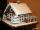Janka and Marienka calculated that there are 210 gingerbreads on the gingerbread house. Janko ate one-seventh of all gingerbreads, and Marienka ate a third less than Janko. How many gingerbreads remained in the gingerbread house?
• ClarissaClarissa read 1/2 of a book in the morning, and she read some more at night. By the end of the day, she still had 1/3 of the book left to read. How much of the book did Clarissa read at night?
• Bus vs train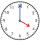Milada took the bus and the journey took 55 minutes. Jarmila was 1h 20 min by train. They arrived in Prague at the same time 10h45 min. At what time did each have to go out?
• There 14There are 250 people in a museum. 2/5 of the 250 people are girls 3/10 of the 250 people are boys The rest of the 250 people are adults Work out the number of adults in the museum.
• Difference of two fractions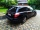What is the difference between 1/2 and 1/6? (Write the answer as a fraction in lowest terms. )
• MichaelMichael had a bar of chocolate. He ate 1/2 of it and gave away 1/3. What fraction had he left?
• IssacIssac eats 1/6 of the pizza. Maya then eats 3/5 of the remaining pizza. What fraction of the original pizza is left?
• Toilets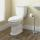Federal law requires that all residential toilets sold in the United States use no more than 1 3/5 gallons of water per flush. Before this legislation, conventional toilets used 3 2/5 gallons of water per flush. Find the amount of water saved in one year
• HusseinHussein owns 450000 crowns (local currency). He spent at the bookstore 2 over 9 to buy some books and tales. He paid 3 over 5 of his money to buy his math book. a. Calculate the remaining amount of money with Hussein? b. Hussein lost 3 over 4 of the remai
• Mrs. SusanMrs. Susan bought 1/8 m of curtain cloth. She used 3/5 m to make a curtain for the living room window. How many meters of cloth were not used?
• A farmer's heritage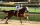A farmer died leaving his 17 horses to his 3 sons. When his sons opened up the Will it read: My eldest son should get 1/2 (half) of total horses; My middle son should be given 1/3rd (one-third) of the total horses; My youngest son should be given 1/9th (o
• Bucket of clay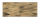Tina and Bill share a 12-ounce bucket of clay. By the end of the week, Tina has used 1/6 of the bucket, and Bill has used 2/3 of the bucket of clay. How many ounces are left in the bucket?
• On day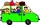On day one of the trip they drove 1/4 of the total distance. On day two they drove 1/2 of the total distance. What fraction of the total distance is left to travel?